# Kaggle系列-Mechanisms of Action (MoA) Prediction[表格数据多标签]第一名方案

+关注继续查看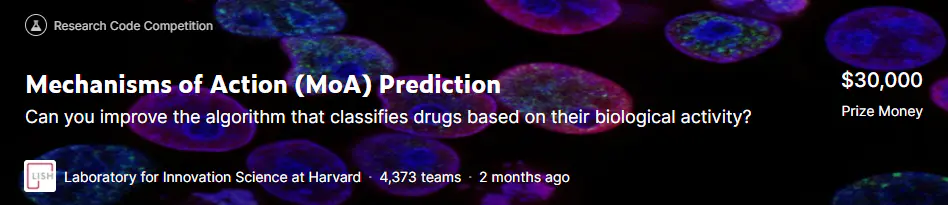## 赛题背景## 赛题任务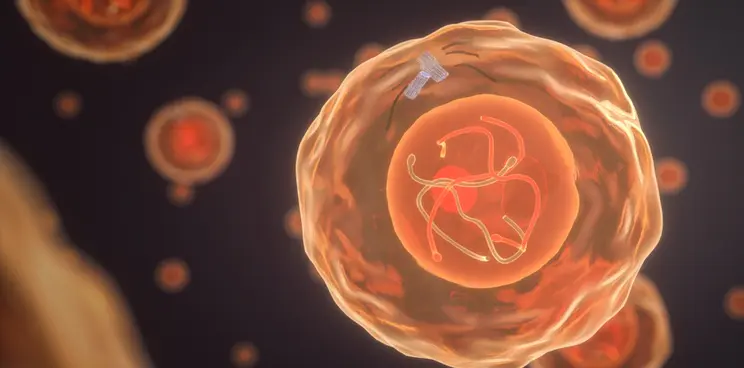## 赛题数据

• train_features.csv:训练集对应的特征数据。特征g-表示基因表达数据，c-表示细胞活力数据。cp_type表示使用化合物(cp_vehicle)或控制扰动(ctrl_vehicle)处理的样品;控制扰动无moa;cp_time和cp_dose表示治疗时间(24、48、72小时)和剂量(高、低)。
• train_drug.csv 训练集样本的sig_id和drug_id对应关系
• train_targets_scored.csv：MOA的二分类标签数据
• train_targets_nonscored.csv：额外没有带有标签的MOA数据
• test_features.csv：测试数据的特征。需要选手预测测试数据中每一行的每个MoA得分的概率。
• sample_submission.csv：提交文件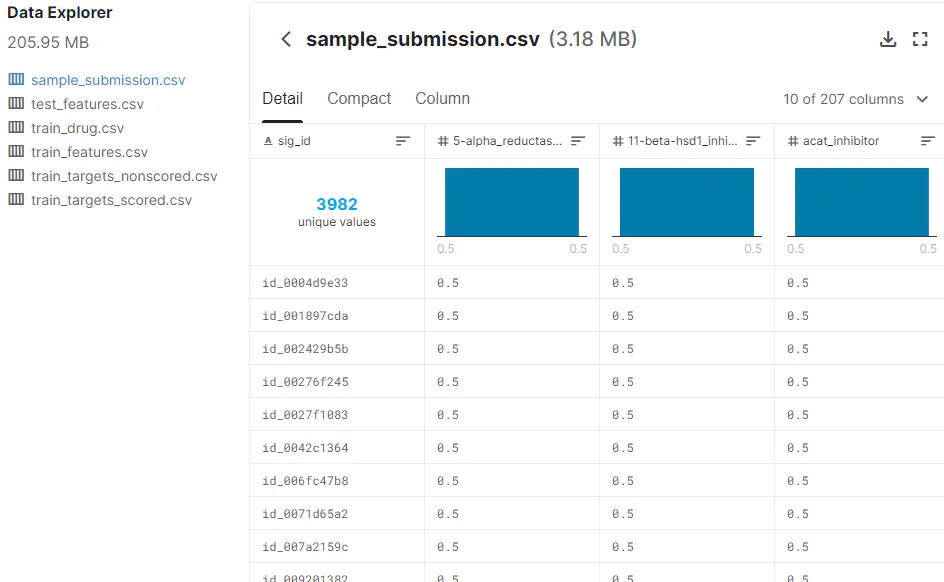## 数据分析

https://www.kaggle.com/isaienkov/mechanisms-of-action-moa-prediction-eda

### 特征数据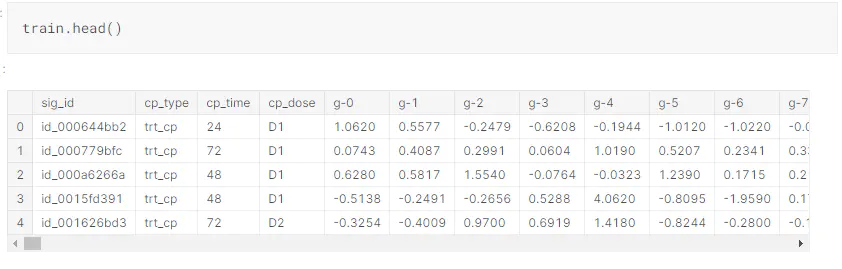Number of rows in training set: 23814
Number of columns in training set: 876
Number of rows in test set: 3982
Number of columns in test set: 876

### 类别特征可视化

• cp_type化合物处理方式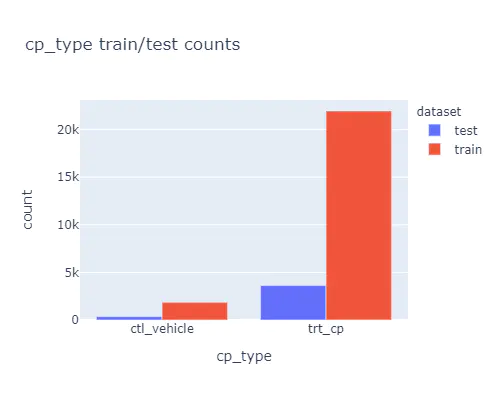• cp_time 实验时间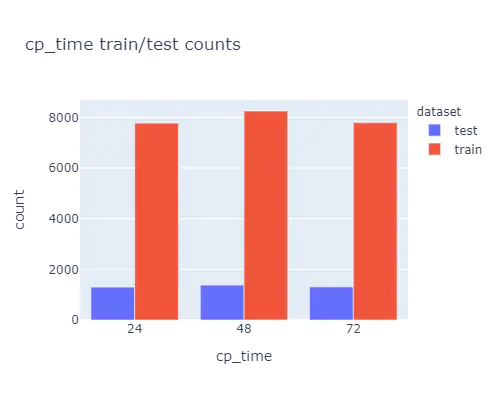• cp_dose 试验剂量ds = df[df['dataset']=='train']
ds = ds.groupby(['cp_type', 'cp_time', 'cp_dose'])['sig_id'].count().reset_index()
ds.columns = [
'cp_type',
'cp_time',
'cp_dose',
'count'
]
fig = px.sunburst(
ds,
path=[
'cp_type',
'cp_time',
'cp_dose'
],
values='count',
title='Sunburst chart for all cp_type/cp_time/cp_dose',
width=500,
height=500
)
fig.show()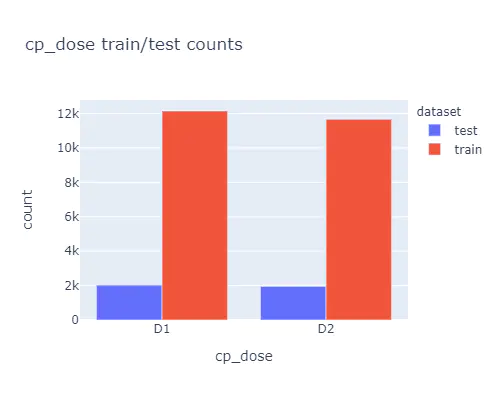### 基因细胞特征分布

g代表gene特征数据，c代表cell特征数据

train_columns = train.columns.to_list()
g_list = [i for i in train_columns if i.startswith('g-')]
c_list = [i for i in train_columns if i.startswith('c-')]

def plot_set_histograms(plot_list, title):
fig = make_subplots(
rows=4,
cols=3
)

traces = [
go.Histogram(
x=train[col],
nbinsx=100,
name=col
) for col in plot_list
]
for i in range(len(traces)):
fig.append_trace(
traces[i],
(i // 3) + 1,
(i % 3) + 1
)
fig.update_layout(
title_text=title,
height=1000,
width=WIDTH
)
fig.show()
plot_list = [
g_list[
np.random.randint(0, len(g_list)-1)
] for i in range(50)
]
plot_list = list(set(plot_list))[:12]
plot_set_histograms(plot_list, 'Randomly selected gene expression features distributions')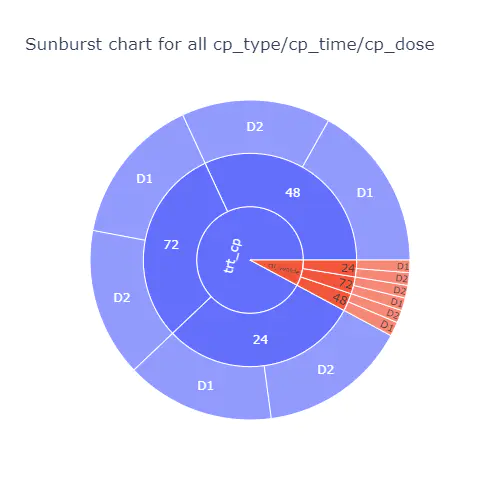### 特征相关性

columns = g_list + c_list
for_correlation = random.sample(columns, 50)
data = df[for_correlation]
f = plt.figure(
figsize=(18, 18)
)
plt.matshow(
data.corr(),
fignum=f.number
)
plt.xticks(
range(data.shape),
data.columns,
fontsize=14,
rotation=50
)
plt.yticks(
range(data.shape),
data.columns,
fontsize=14
)
cb = plt.colorbar()
cb.ax.tick_params(
labelsize=13
)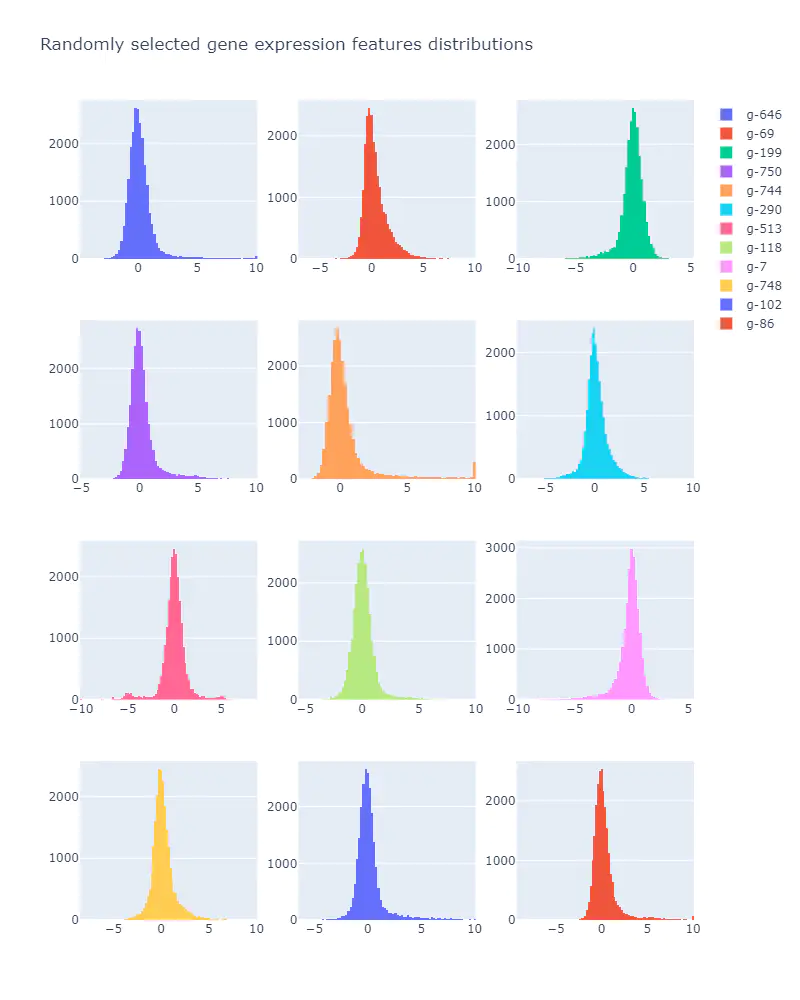### 目标分析

correlation_matrix['train_features'] = columns
correlation_matrix = correlation_matrix.set_index('train_features')
correlation_matrix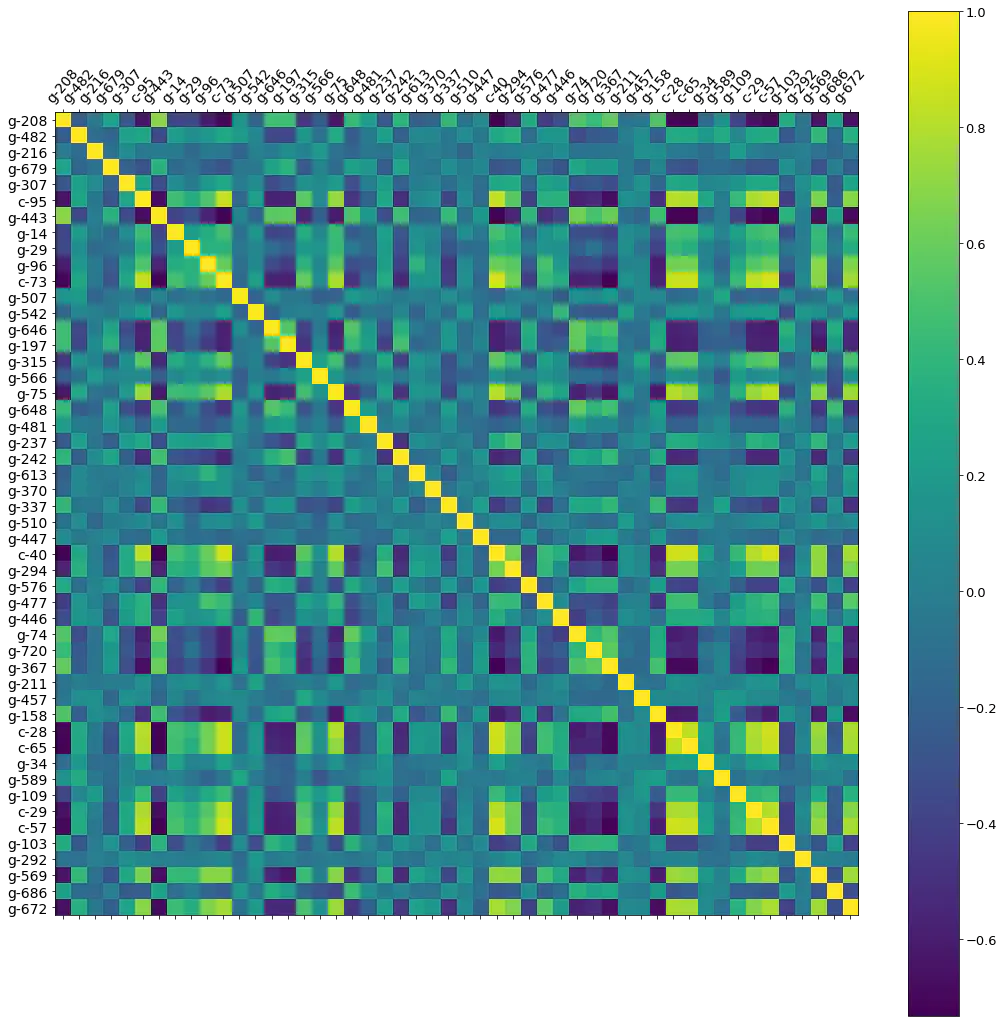count_features = total_scores['train_best_column'].value_counts().reset_index().sort_values('train_best_column')
count_features.columns = [
'column',
'count'
]
count_features = count_features.tail(33)
fig = px.bar(
count_features,
x='count',
y="column",
orientation='h',
title='Columns from training set with number of high correlations with target columns',
width=WIDTH,
height=700
)
fig.show()• 二阶相关性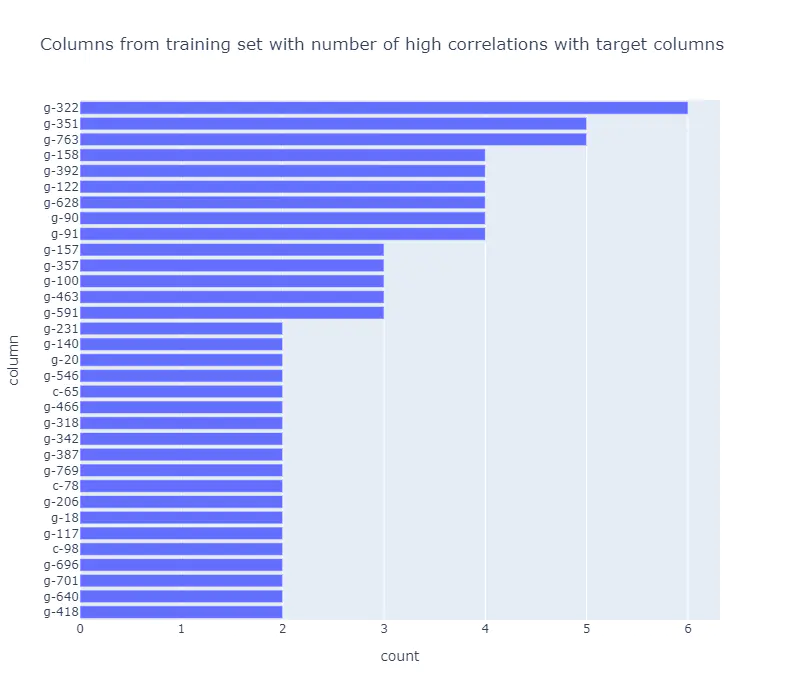• 三阶相关性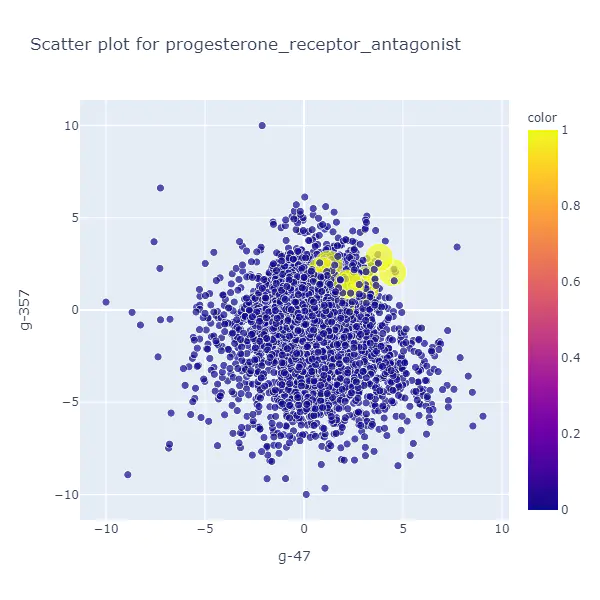## Hungry for Gold 第一名方案

### 模型1 3-Stage NN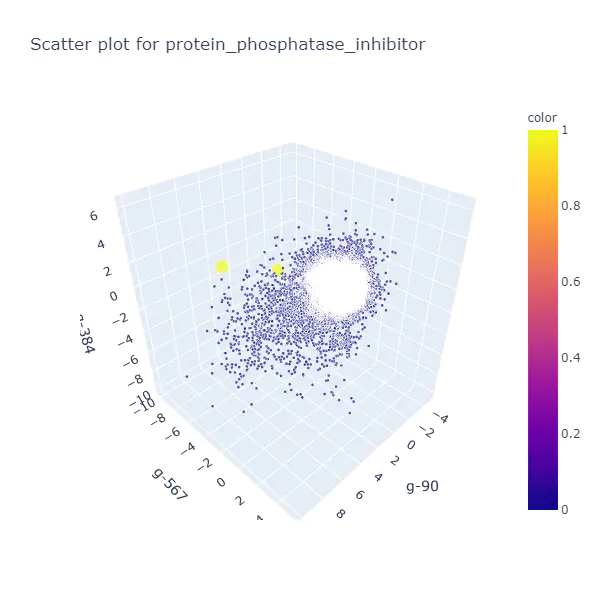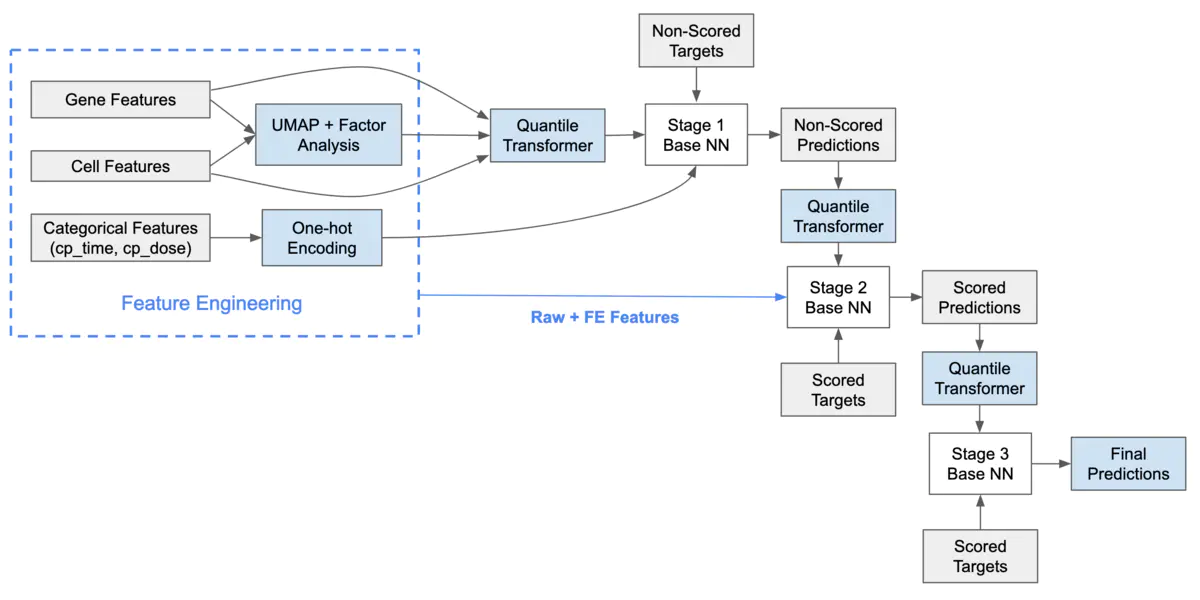### 模型2 2-Stage NN+TabNet

dreamquark-ai/tabnet https://github.com/dreamquark-ai/tabnet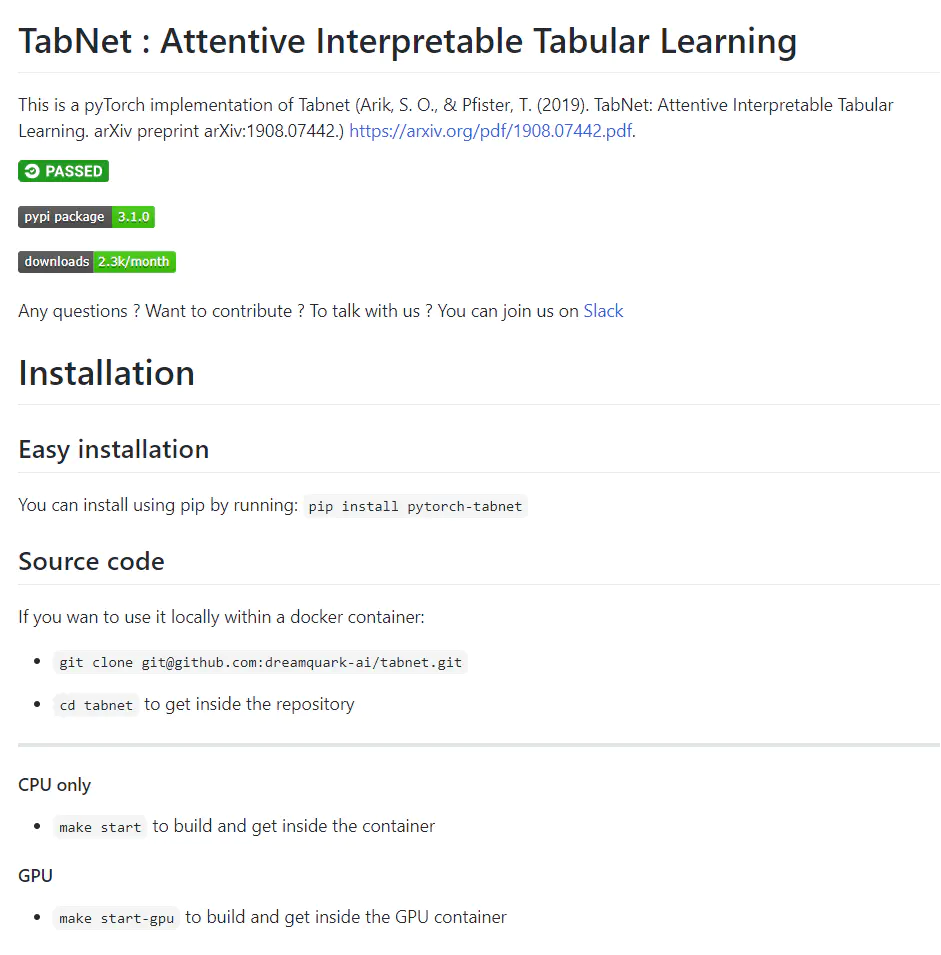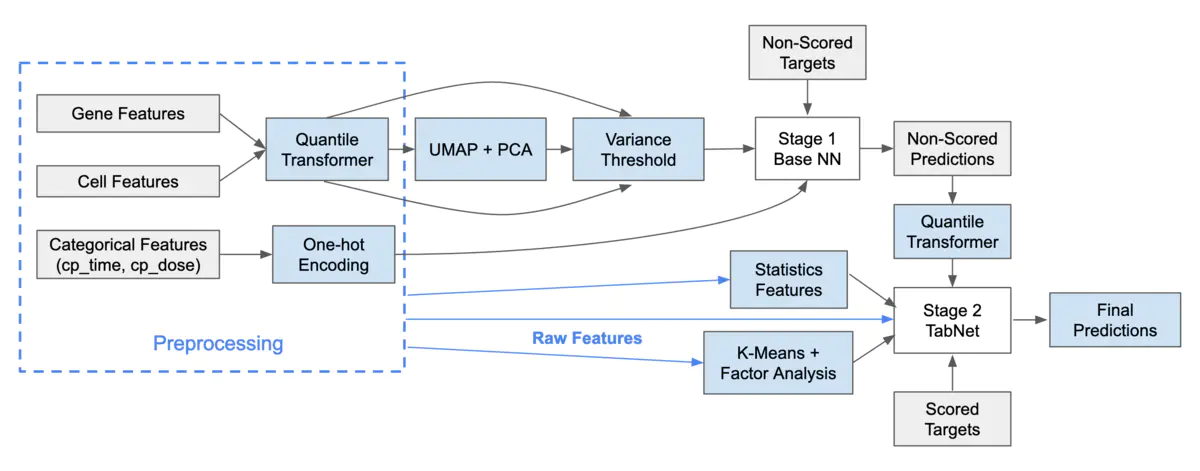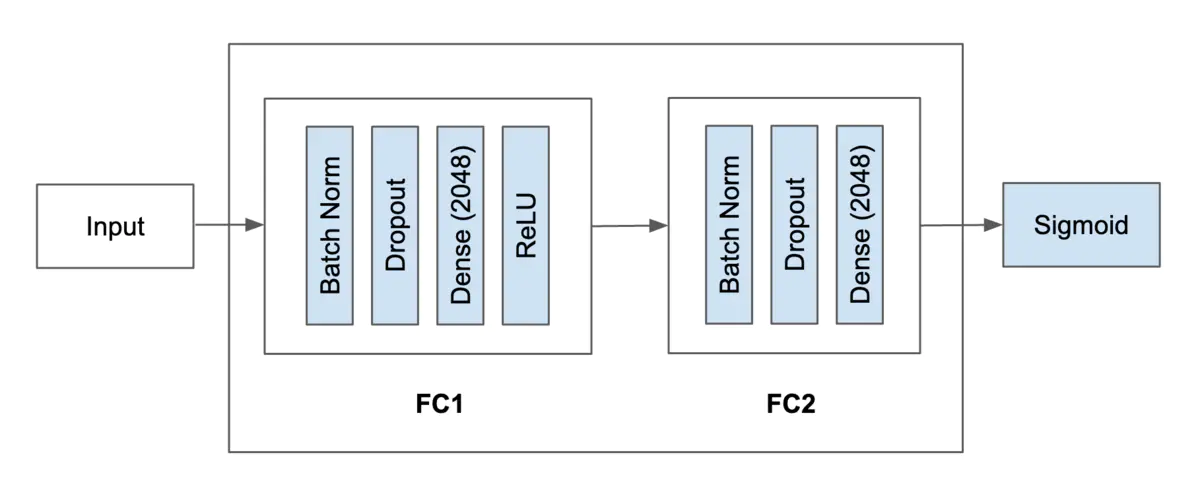### 模型3 Simple NN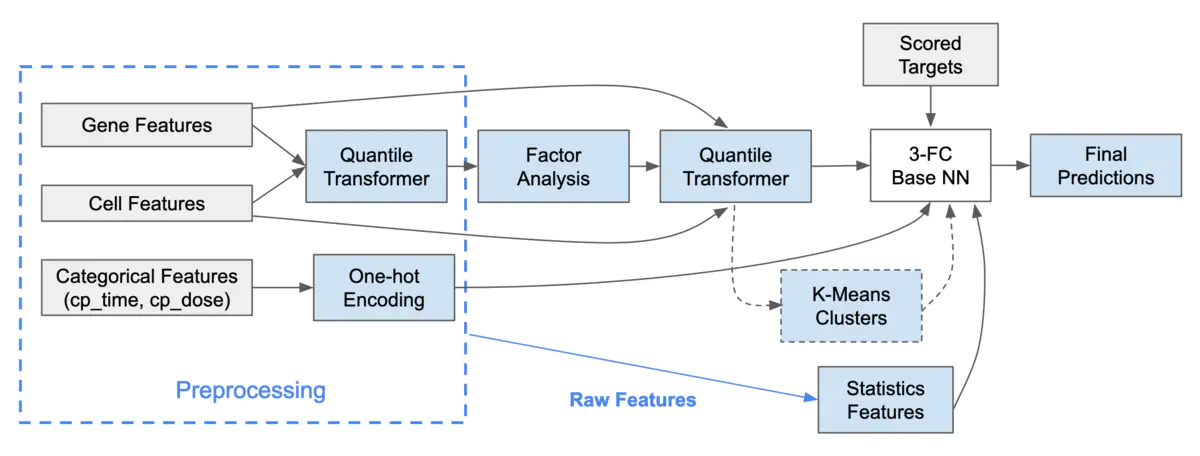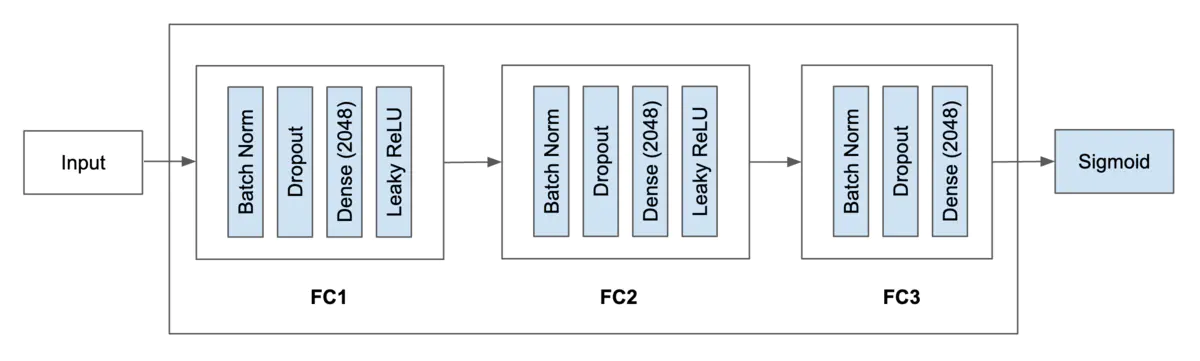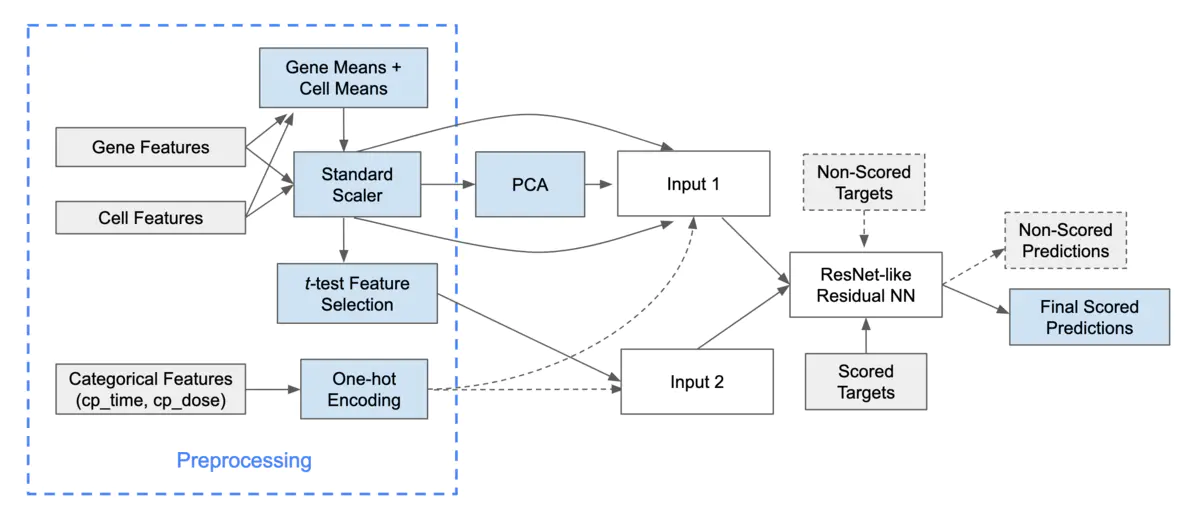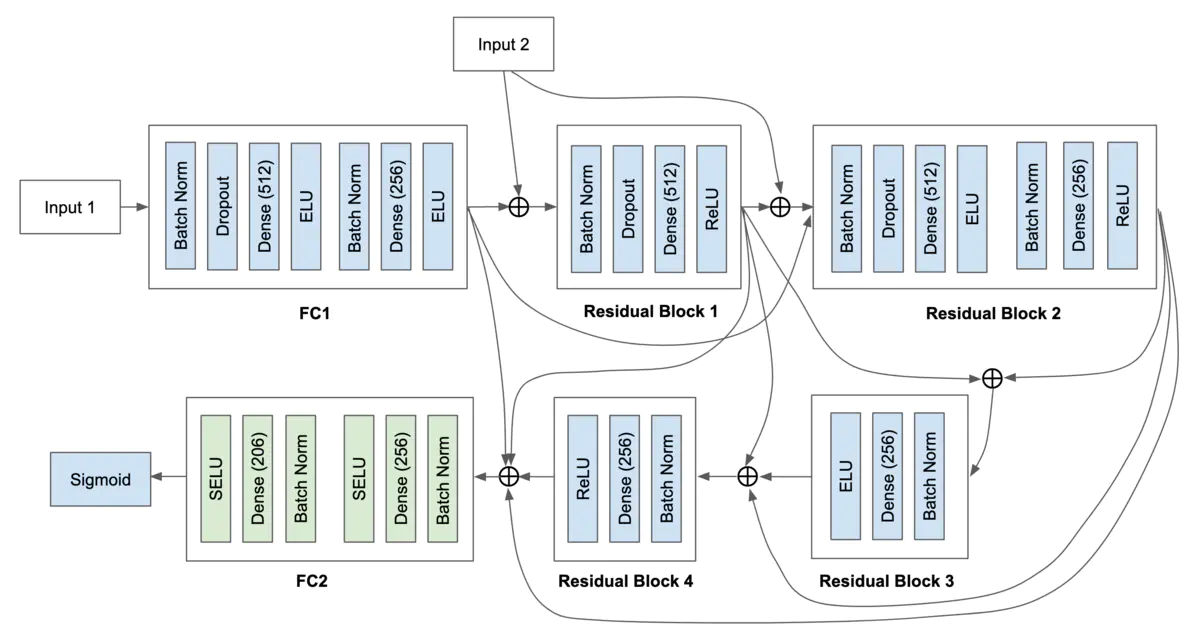### 模型5 DeepInsight CNNs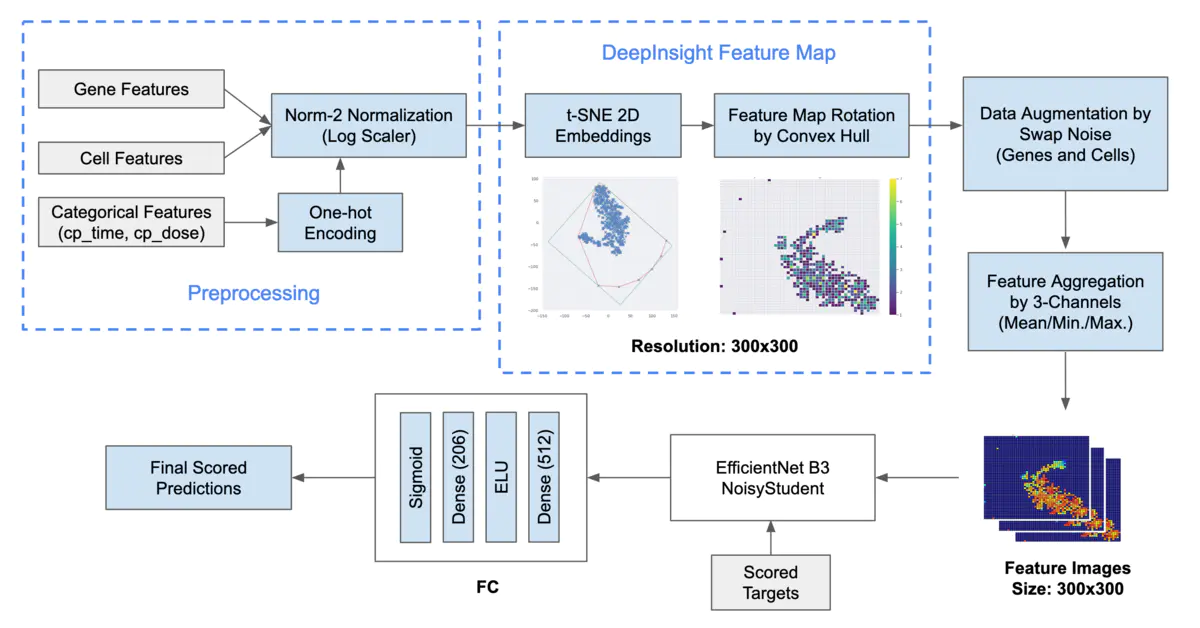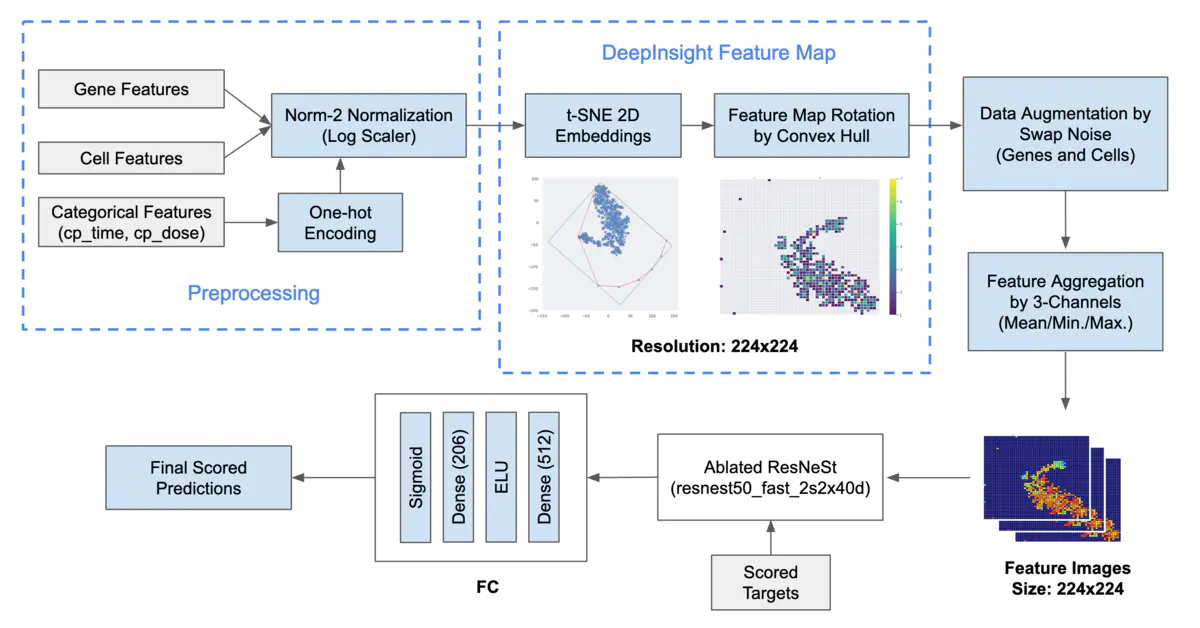### 特征多样性

• 基于线上提交得出的模型相关性热力图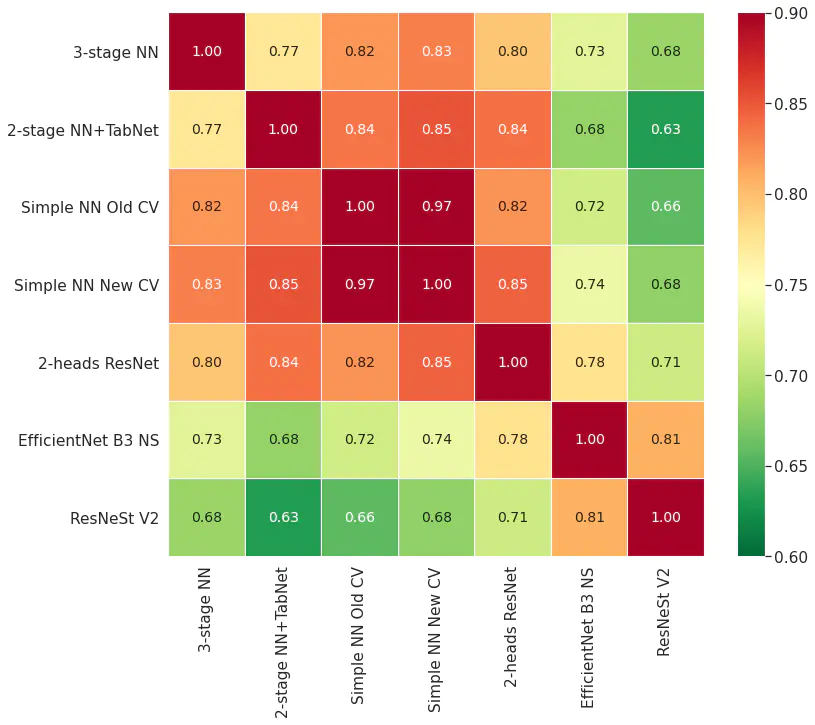• 基于线下OOF得出的模型相关性热力图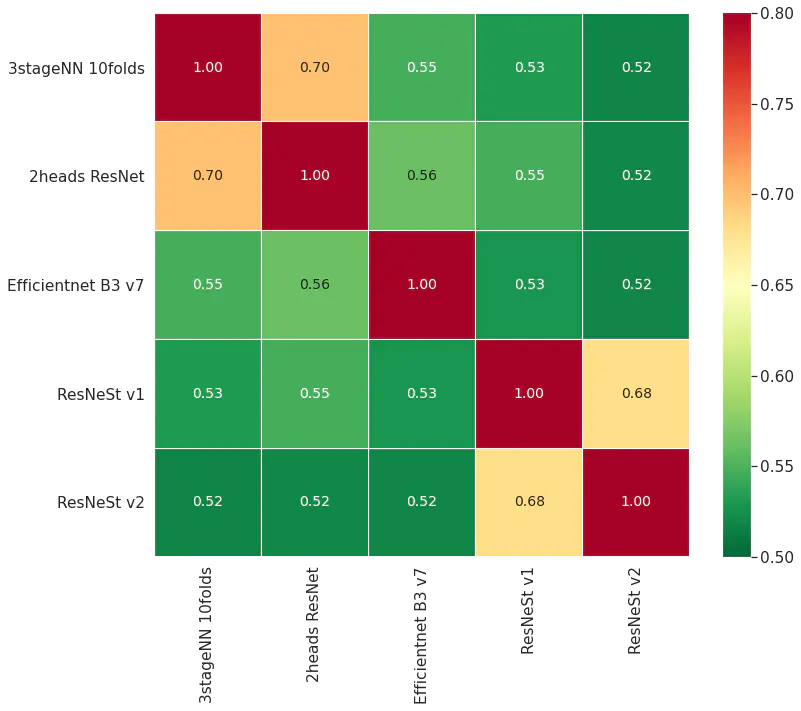可以看到模型相关性系数都很低，一般在0.52-0.70，模型具有多样性和差异性，那么融合之后的收益会很大

## 总结

• 本方案使用神经网络在白表格数据上做了大量尝试，并取得很大的成效
• 为了提高模型融合的收益，需要保证模型多样性，所以作者也使用了不同的训练方法和模型结构
• 使用CNN等预训练模型提取特征图19764 063945 029066 022526 0Xshell使用SSH远程登录阿里云ECS服务器CentOS7
22305 0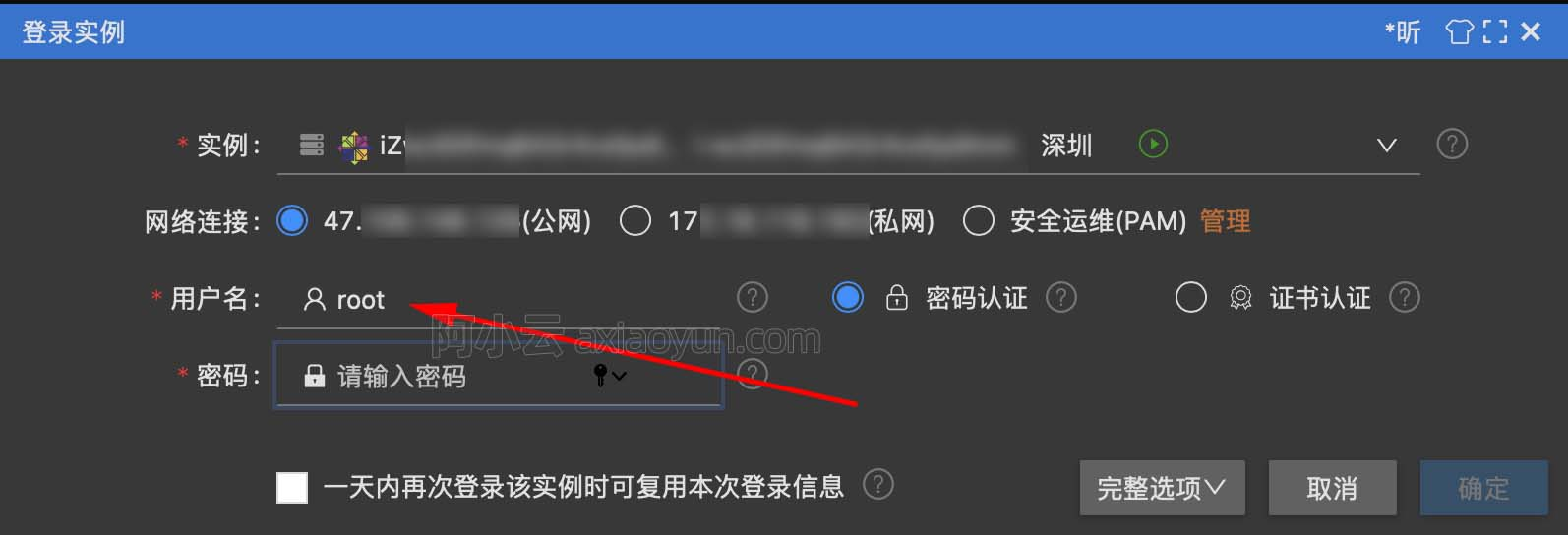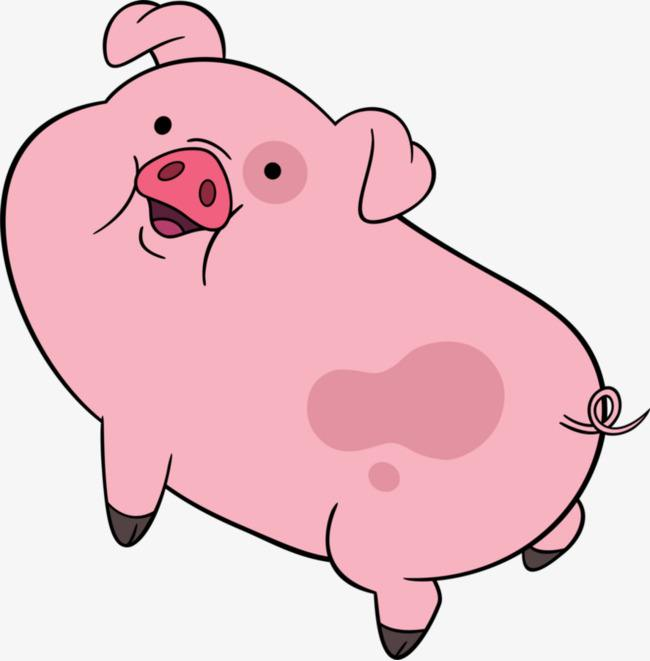16411 015936 020682 023576 014896 0
400

0

JS零基础入门教程（上册）